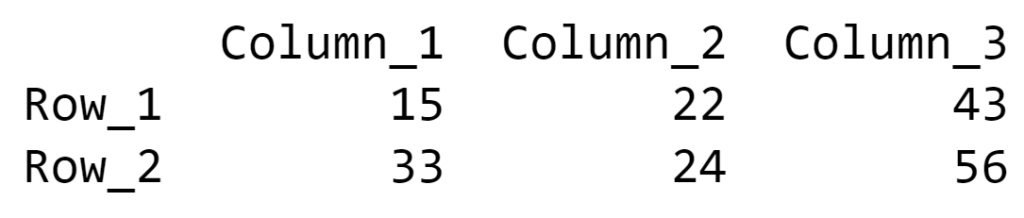# Create a Pandas DataFrame from a Numpy array and specify the index column and column headers

This article demonstrates multiple examples to convert the Numpy arrays into Pandas Dataframe and to specify the index column and column headers for the data frame.

Example 1: In this example, the Pandas dataframe will be generated and proper names of index column and column headers are mentioned in the function. This approach can be used when there is no pattern in naming the index column or column headers.

Below is the implementation:

## Python3

 `# Python program to Create a  ` `# Pandas DataFrame from a Numpy   ` `# array and specify the index  ` `# column and column headers ` ` `  `# import required libraries ` `import` `numpy as np ` `import` `pandas as pd ` ` `  `# creating a numpy array ` `numpyArray ``=` `np.array([[``15``, ``22``, ``43``],  ` `                       ``[``33``, ``24``, ``56``]]) ` ` `  `# generating the Pandas dataframe ` `# from the Numpy array and specifying ` `# name of index and columns ` `panda_df ``=` `pd.DataFrame(data ``=` `numpyArray,  ` `                        ``index ``=` `[``"Row_1"``, ``"Row_2"``],  ` `                        ``columns ``=` `[``"Column_1"``, ` `                                   ``"Column_2"``, ``"Column_3"``]) ` ` `  `# printing the dataframe ` `print``(panda_df) `

Output:Example 2: In this example, the index column and column headers are generated through iteration. The range of iterations for rows and columns are defined by the shape of the Numpy array. With every iteration, a digit will be added to the predefined string and the new index column or column header will generate. Thus, if there is some pattern in naming the labels of the dataframe this approach is suitable.

Below is the implementation:

## Python3

 `# Python program to Create a  ` `# Pandas DataFrame from a Numpy   ` `# array and specify the index column  ` `# and column headers ` ` `  `# import required libraries ` `import` `pandas as pd ` `import` `numpy as np ` ` `  `# creating a numpy array ` `numpyArray ``=` `np.array([[``15``, ``22``, ``43``],  ` `                       ``[``33``, ``24``, ``56``]]) ` ` `  `# generating the Pandas dataframe ` `# from the Numpy array and specifying ` `# name of index and columns ` `panda_df ``=` `pd.DataFrame(data ``=` `numpyArray[``0``:, ``0``:], ` `                        ``index ``=` `[``'Row_'` `+` `str``(i ``+` `1``)  ` `                        ``for` `i ``in` `range``(numpyArray.shape[``0``])], ` `                        ``columns ``=` `[``'Column_'` `+` `str``(i ``+` `1``)  ` `                        ``for` `i ``in` `range``(numpyArray.shape[``1``])]) ` ` `  `# printing the dataframe ` `print``(panda_df) `

Output:Example 3: In this example, the index column and column headers are defined before converting the Numpy array into Pandas dataframe. The label names are again generated through iterations but the method is little different. Here, the number of iterations is defined by the length of the sub-array inside the Numpy array. This method can be used if the index column and column header names follow some pattern.

Below is the implementation:

## Python3

 `# Python program to Create a  ` `# Pandas DataFrame from a Numpy   ` `# array and specify the index column  ` `# and column headers ` ` `  `# import required libraries ` `import` `pandas as pd ` `import` `numpy as np ` ` `  `# creating a numpy array ` `numpyArray ``=` `np.array([[``15``, ``22``, ``43``],  ` `                       ``[``33``, ``24``, ``56``]]) ` ` `  `# defining index for the  ` `# Pandas dataframe ` `index ``=` `[``'Row_'` `+` `str``(i)  ` `        ``for` `i ``in` `range``(``1``, ``len``(numpyArray) ``+` `1``)] ` ` `  `# defining column headers for the  ` `# Pandas dataframe ` `columns ``=` `[``'Column_'` `+` `str``(i)  ` `          ``for` `i ``in` `range``(``1``, ``len``(numpyArray[``0``]) ``+` `1``)] ` ` `  `# generating the Pandas dataframe ` `# from the Numpy array and specifying ` `# details of index and column headers ` `panda_df ``=` `pd.DataFrame(numpyArray ,  ` `                        ``index ``=` `index, ` `                        ``columns ``=` `columns) ` ` `  `# printing the dataframe ` `print``(panda_df) `

Output:Example #4: In this approach, the index column and the column headers for the Pandas dataframe will present itself in the Numpy array. During the conversion of the Numpy array into Pandas data frame, proper indexing for the sub-arrays of the Numpy array has to be done in order to get correct sequence of the dataframe labels.

Below is the implementation:

## Python3

 `# Python program to Create a  ` `# Pandas DataFrame from a Numpy   ` `# array and specify the index column  ` `# and column headers ` ` `  `# import required libraries ` `import` `pandas as pd ` `import` `numpy as np ` ` `  `# creating a numpy array and ` `# specifying the index and  ` `# column headers along with  ` `# data stored in the array ` `numpyArray ``=` `np.array([['``', '``Column_1',  ` `                       ``'Column_2'``, ``'Column_3'``], ` `                       ``[``'Row_1'``, ``15``, ``22``, ``43``], ` `                       ``[``'Row_2'``, ``33``, ``24``, ``56``]]) ` ` `  `# generating the Pandas dataframe ` `# from the Numpy array and specifying ` `# details of index and column headers ` `panda_df ``=` `pd.DataFrame(data ``=` `numpyArray[``1``:, ``1``:], ` `                        ``index ``=` `numpyArray[``1``:, ``0``], ` `                        ``columns ``=` `numpyArray[``0``, ``1``:]) ` ` `  `# printing the dataframe ` `print``(panda_df) `

Output:My Personal Notes arrow_drop_up3rd Year BE CSE Student, Loves coding, Patience and hardworking guy, Ready for challenges and for learning new things

If you like GeeksforGeeks and would like to contribute, you can also write an article using contribute.geeksforgeeks.org or mail your article to contribute@geeksforgeeks.org. See your article appearing on the GeeksforGeeks main page and help other Geeks.

Please Improve this article if you find anything incorrect by clicking on the "Improve Article" button below.

Article Tags :

1

Please write to us at contribute@geeksforgeeks.org to report any issue with the above content.